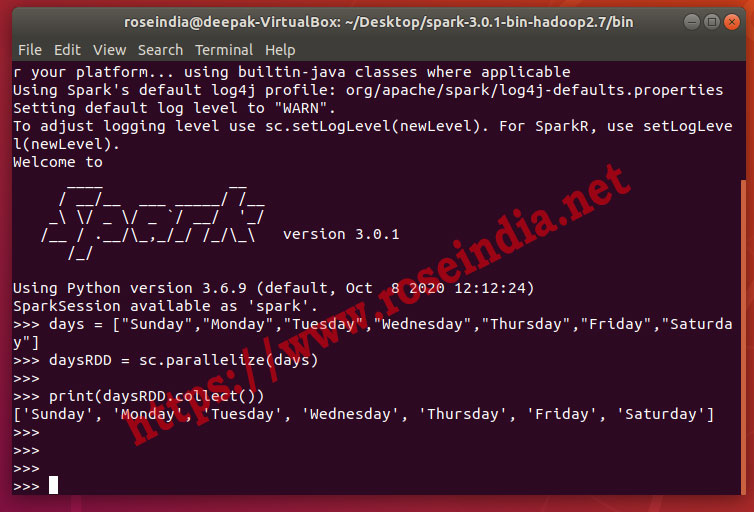Tutorials

# sc.parallelize pyspark - use of sc.parallelize in pyspark program

In this section we are going to provide you examples of using the sc.parallelize function in PySpark.

In this section we are going to provide you examples of using the sc.parallelize function in PySpark.

## sc.parallelize - How do you parallelize in PySpark?

In this tutorial we will understand the use of sc.parallelize in PySpark and see how to parallelize in PySpark? The parallelize of SparkContext is used to create distributed dataset which can be distributed on the worker nodes and used for distributed processing in Spark. The sc.parallelize method is used to create parallelize collection of RDD from a list of collection. For example if you have a list which contains values you can use the sc.parallelize method to convert into RDD which can be distributed over cluster for parallel processing. In this tutorial we are going to learn many example of using the sc.parallelize  method for creating the RDD in PySpark program.

In this section we will show examples to parallelize:

• String collection
• Integer collection

Let's see the examples of sc.parallelize in PySpark.

Parallelize String collection in PySpark

In this following we are going to create a list of string and then use the sc.parallelize method to parallelize the data into RDD. Here is example code:

``````
days = ["Sunday","Monday","Tuesday","Wednesday","Thursday","Friday","Saturday"]
daysRDD = sc.parallelize(days)

print(daysRDD.collect())
``````

Here is the screen shot of program execution:Parallelize Integer collection in PySpark

Now we  will show you how you can parallelize integer collection in PySpark. Here is the code example:

``````
# Parallelize number array

numberArray = [1,2,3,4,5,6,7,8,9,10]

numbersRDD = sc.parallelize(numberArray)

print(numbersRDD.collect())

# perform sum with reduce
sumTotal = numbersRDD.reduce(lambda a, b: a+b)

# print type of variable
type(sumTotal)

# Print total
print(sumTotal)
``````

Following is the output of running the above code:The SparkContext parallelize() method is used to create RDD from the collection objet and in the above examples we have shown you the examples of creating RDD from String and Integer collection. With the help of SparkContext parallelize() method you can easily create RDD which is distributed on the spark worker nodes and run any other distributed processing. Apache Spark is powerful framework for running data processing workloads on the distributed cluster.

In the above example we have first created the collection object with values inside it then used the parallelize() method of SparkContext to create RDD from the data. The parallelize() method takes collection object as parameter and then convert it into RDD. The RDD is distributed data set and it is distributed on the available nodes in the Spark cluster.

The parallelize() method is part of Spark Driver and it runs on the driver node. On the driver node it runs which takes list of objects and converts into distributed RDD data sets. The parallelize() method creates a new RDD and distribute the data on the worker nodes. On the worker nodes RDD can be processed based on the program written by developers. Developers should learn parallelize() method of Apache Spark in detail and this tutorial showed you examples of creating RDD with parallelize() method of SparkContext.

Related tutorials: